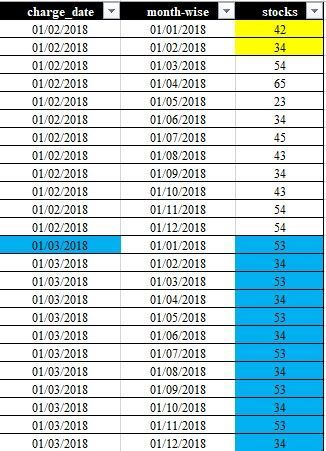cancel
Showing results for
Did you mean:Helper III

## DAX help

I have charge date (which is first day of each month) as column , and month wise column (first day of each month ) and stocks column.

i need if i select one month in date slicer (charge date) for example(01/03/2018) .

it should show future stocks value from stock column from 01/03/2018(month wise column) to 01/12/2018 (month wise column). (blue colour mentioned in future stock)

and it should show actual stock value from stock column - previous month of (month wise column) (01/01/2018 and 01/02/2018)(blue colour mentioned in actual stock )

and it dynamically take the values from previous month of selected charge date for future stock (mentioned in yellow colour )

i want to show it in line chart (actual stock and future stock).

The data is attachedthe desired output i need is1 ACCEPTED SOLUTIONCommunity Support

You can create two measures:

``````Actual_ = IF(SELECTEDVALUE('Table (3)'[month-wise])<SELECTEDVALUE('Table (3)'[charge_date]),SUM([stocks]),0)

Future_ = IF(SELECTEDVALUE('Table (3)'[month-wise])>=SELECTEDVALUE('Table (3)'[charge_date]),SUM([stocks]),SUMX(FILTER(ALL('Table (3)'),'Table (3)'[month-wise]=SELECTEDVALUE('Table (3)'[month-wise])&&'Table (3)'[charge_date]=SELECTEDVALUE('Table (3)'[charge_date])-1),[stocks]))``````Best Regards,

Yolo Zhu

If this post helps, then please consider Accept it as the solution to help the other members find it more quickly.

2 REPLIES 2Community Support

You can create two measures:

``````Actual_ = IF(SELECTEDVALUE('Table (3)'[month-wise])<SELECTEDVALUE('Table (3)'[charge_date]),SUM([stocks]),0)

Future_ = IF(SELECTEDVALUE('Table (3)'[month-wise])>=SELECTEDVALUE('Table (3)'[charge_date]),SUM([stocks]),SUMX(FILTER(ALL('Table (3)'),'Table (3)'[month-wise]=SELECTEDVALUE('Table (3)'[month-wise])&&'Table (3)'[charge_date]=SELECTEDVALUE('Table (3)'[charge_date])-1),[stocks]))``````Best Regards,

Yolo Zhu

If this post helps, then please consider Accept it as the solution to help the other members find it more quickly.Helper III

@v-xinruzhu-msft @hi ,great hatsoff for your solution. I need difference between actuals and future stocks in seperate column as difference. but that difference should be upto selected charge date (for example ,if I select 1/3/2018 in charge date ,difference column should show upto previous month 1/2/2018 ).please help me out